198. 打家劫舍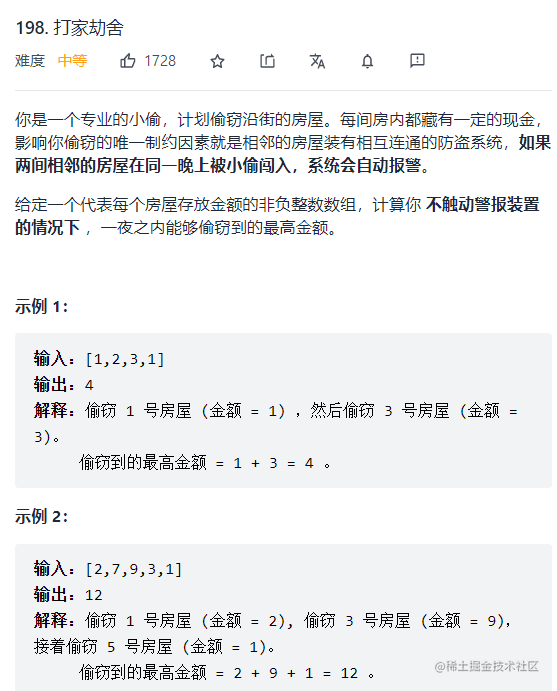public int rob(int[] nums);

• 如果偷，那么前一间屋n-1一定没有偷，我们考虑n-2之前的最优选择，加上n就可以了；
• 如果不偷，那么n-1之前的最优选择，就是当前的最优选择。

• 如果你抢了这间房子，那么你肯定不能抢相邻的下一间房子了，只能从下下间房子开始做选择。

• 如果你不抢这间房子，那么你可以走到下一间房子前，继续做选择。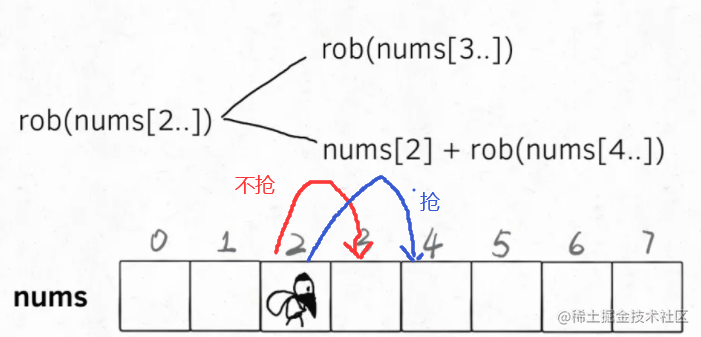dp[i] = Math.max(dp[i-1], dp[i-2] + nums[i])

class Solution {
public int rob(int[] nums) {
int n = nums.length;
if(n == 1) {
return nums;
}
//定义状态，
int[] dp = new int[n];
dp = nums;
dp = Math.max(dp, nums);
for(int i = 2; i < n; i++){
dp[i] = Math.max(dp[i-1], dp[i-2] + nums[i]);
}
return dp[n-1];
}
}

class Solution {
public int rob(int[] nums) {
int n = nums.length;
if(n == 1) {
return nums;
}
//定义状态，
int pre1 = nums;
int pre2 = Math.max(pre1, nums);
for(int i = 2; i < n; i++){
int temp = Math.max(pre2, pre1 + nums[i]);
pre1 = pre2;
pre2 = temp;
}
return pre2;
}
}

213. 打家劫舍 II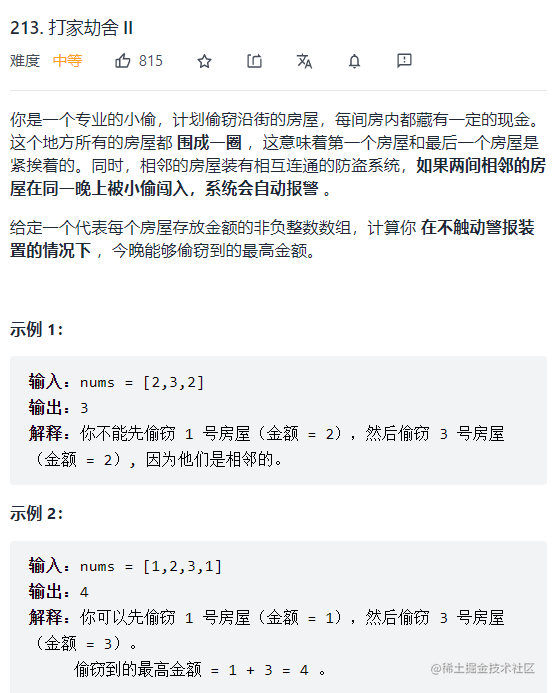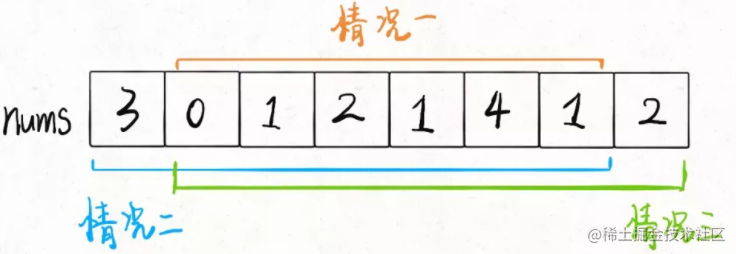class Solution {
public int rob(int[] nums) {
int n = nums.length;
if(n == 1) {
return nums;
}
return Math.max(robRange(nums, 0, n-2), robRange(nums, 1, n-1));
}

public int robRange(int[] nums, int start, int end) {
if(start == end) {
return nums[start];
}
//定义状态，
int pre1 = nums[start];
int pre2 = Math.max(pre1, nums[start + 1]);
for(int i = start + 2; i <= end; i++){
int temp = Math.max(pre2, pre1 + nums[i]);
pre1 = pre2;
pre2 = temp;
}
return pre2;
}
}

337. 打家劫舍 III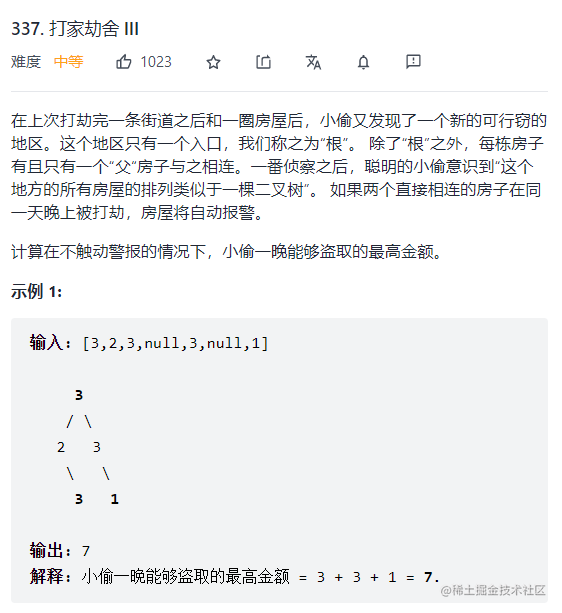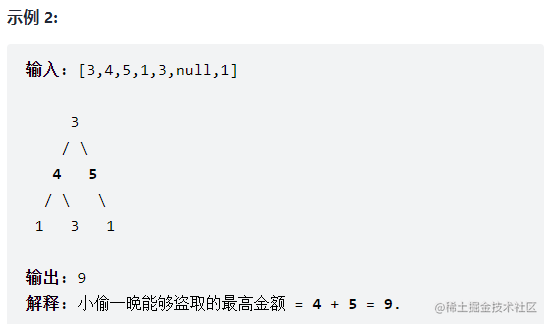解法一、暴力递归 - 最优子结构

4 个孙子偷的钱 + 爷爷的钱 VS 两个儿子偷的钱 哪个组合钱多，就当做当前节点能偷的最大钱数。这就是动态规划里面的最优子结构

• 4 个孙子投的钱加上爷爷的钱如下

int method1 = root.val + rob(root.left.left) + rob(root.left.right) + rob(root.right.left) + rob(root.right.right)

• 两个儿子偷的钱如下

int method2 = rob(root.left) + rob(root.right);

• 挑选一个钱数多的方案则

int result = Math.max(method1, method2);

public int rob(TreeNode root) {
if (root == null) {
return 0;
}

int money = root.val;

if (root.left != null) {
//当前节点加上左边孙子节点的钱
money += (rob(root.left.left) + rob(root.left.right));
}

if (root.right != null) {
//当前节点加上右边孙子节点的钱
money += (rob(root.right.left) + rob(root.right.right));
}

return Math.max(money, rob(root.left) + rob(root.right));
}

解法二、记忆化 - 解决重复子问题

public int rob(TreeNode root) {
HashMap<TreeNode, Integer> memo = new HashMap<>();
return robInternal(root, memo);
}

public int robInternal(TreeNode root, HashMap<TreeNode, Integer> memo) {
if (root == null) {
return 0;
}

if (memo.containsKey(root)) {
return memo.get(root);
}

int money = root.val;

if (root.left != null) {
money += (robInternal(root.left.left, memo) + robInternal(root.left.right, memo));
}
if (root.right != null) {
money += (robInternal(root.right.left, memo) + robInternal(root.right.right, memo));
}
int result = Math.max(money, robInternal(root.left, memo) + robInternal(root.right, memo));
memo.put(root, result);
return result;
}